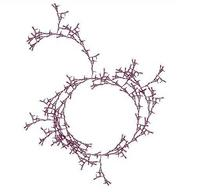University of Virginia, Department of Computer Science CS200: Computer Science, Spring 2003

Notes: Friday 11 April 2003

• Saturday-Wednesday: Design reviews
• Friday, 18 April: Exam 2 out
• Wednesday, 23 April: Exam 2 Due
• 28 April (9am): Problem Set 8 Due

Notes
Universal Turing Machine

A Turing Machine that can simulate any other Turing Machine on an input: TMU (P, I) = the output of running TM-P on input I

What is a calculus?

What properties make Scheme too complex to be a good model of computation?

Lambda Calculus is a set of rules for manipulating symbols. They can be given meanings that map well to computation.

Lambda Calculus Term Grammar

term ::= variable
term ::= term term
term ::= ( term )
term ::= λ variable . term

Rules

Alpha Reduction: (renaming variables)
λ y . Mα λ v . M [y |→ v]) where v does not occur in M.
We can change the name of a lambda variable by replacing all occurances of the variable in the body term with a new name that does not appear in the body term.

Beta Reduction: (substitution)

x . M) Nβ M [ x |→ N ]
All computation can be modeled using Beta Reduction!
Uninteresting Reduction Rules

MM
PMPN if MN
MPNP if MN
λ x . M ⇒ λ x . N ⇒ if MN
M ⇒ P if MN and N ⇒ P

Example

λ f . ((λ x . f (xx)) (λ x . f (xx)))CS 200: Computer Science Department of Computer Science University of Virginia Circle Fractal by Ramsey Arnaoot and Qi Wang cs200-staff@cs.virginia.edu Using these Materials